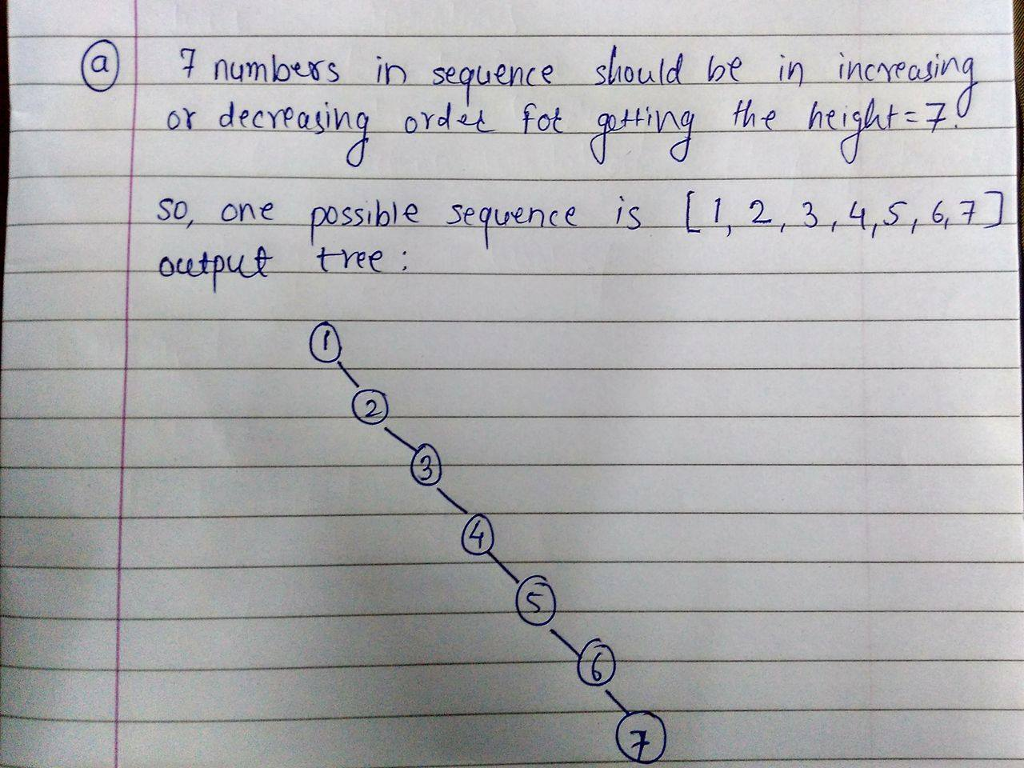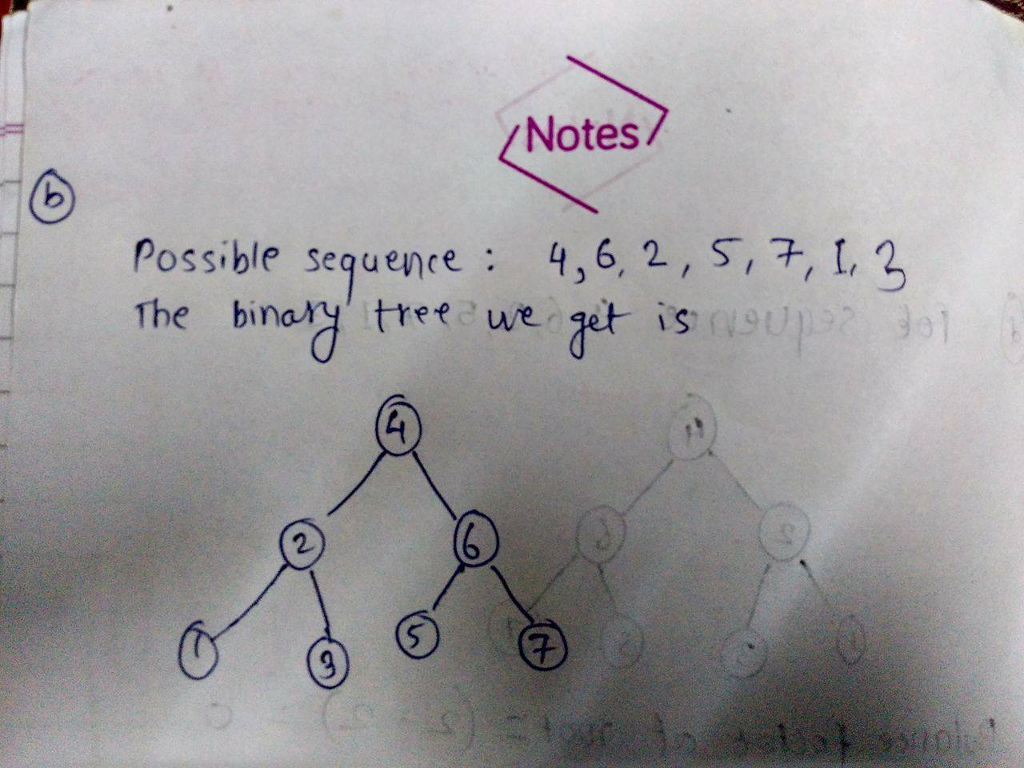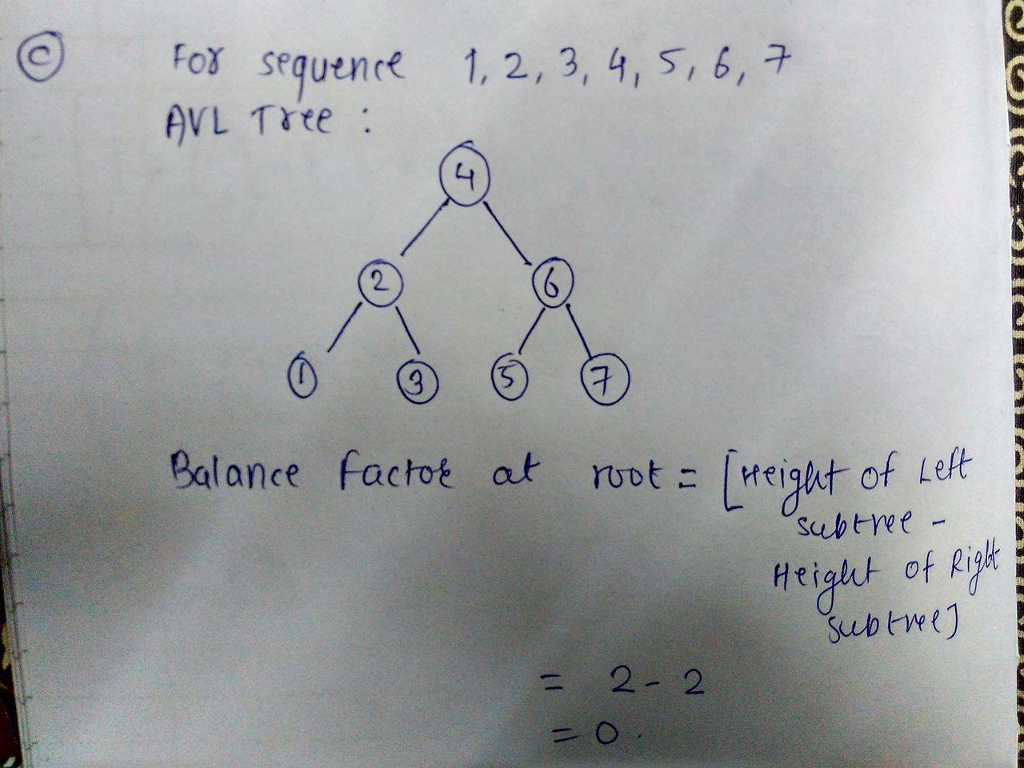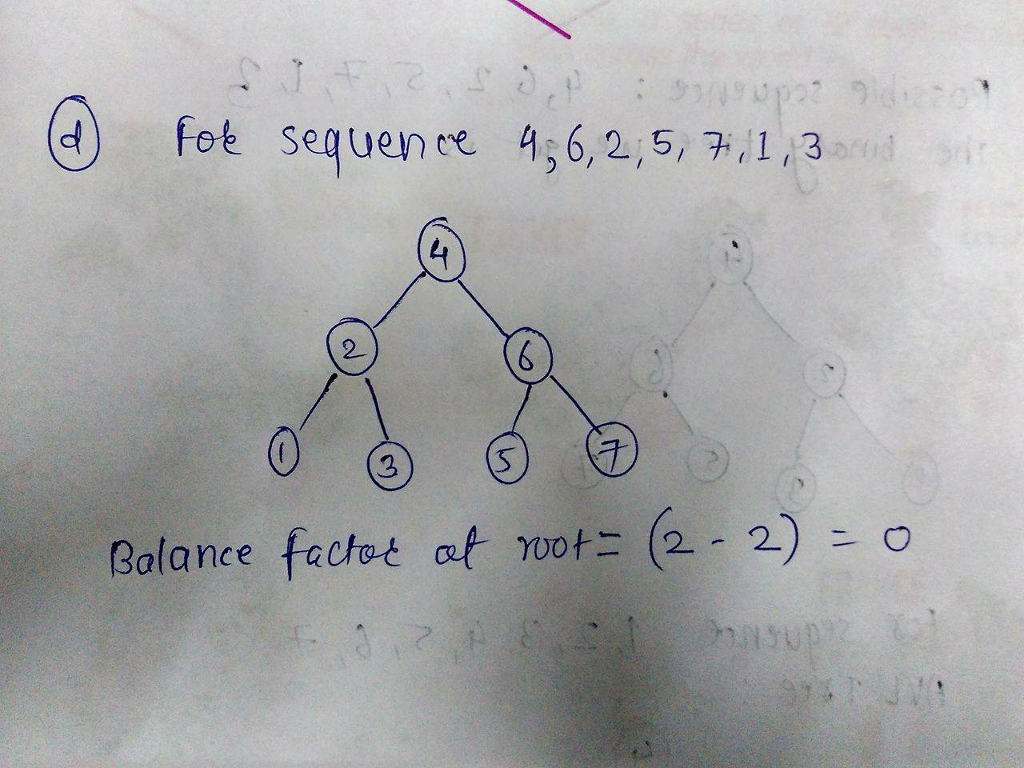# Question & Answer: Select 7 unique integers from 1 to 100. Assume you have an initially empty binary search tree. a……

Select 7 unique integers from 1 to 100. Assume you have an initially empty binary search tree. a. Give a sequence of the selected integers such that when they are inserted into the binary search tree, the resulting tree height is 7. b. Give a sequence of the selected integers such that when they are inserted into the binary search tree, the resulting tree height is 3. c. Using the sequence you gave in part a, insert the numbers into an initially empty AVL tree. Draw the resulting tree and give the balance factor at the root node. d. Using the sequence you gave in part b, insert the numbers into an initially empty AVL tree. Draw the resulting tree and give the balance factor at the root node.